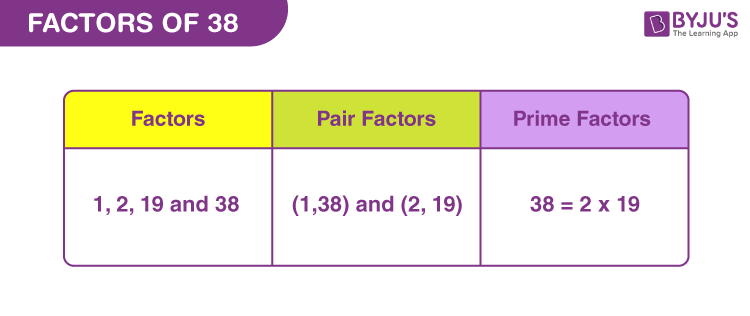# Factors of 38

Factors of 38 are the real numbers that divide the original number, evenly. When 38 is divided by its factor, then the resultant quotient is a whole number and there is no remainder left. As we know 38 is a composite number, it will have factors more than two.

Multiples are generated when 38 is multiplied by consecutive natural numbers. Hence, multiples and factors are different in meaning. But 38 is both a factor and multiple of itself, since,

38 x 1 = 38 [Multiple]

38/38 = 1 [Factor]

Now let us learn to find the factors of 38 along with pair factors and prime factors.## How to Find Factors of 38?

Factors of 38 are the integers that can divide the original number, wholly. Now to find the factors, we need to check by which numbers, 38 is completely divisible. Let us start dividing 38 by the smallest natural number, 1.

38 ÷ 1 = 38

38 ÷ 2 = 19

38 ÷ 19 = 2

38 ÷ 38 = 1

Hence, we can conclude that 1, 2, 19 and 38 are the factors of 38.

### Factors of -38

The factors of -38 will be additive inverse of natural numbers, i.e. negative numbers because dividing -38 by any negative number will result in a positive value.

• (-38) ÷ (-1) = 38
• (-38) ÷ (-2) = 19
• (-38) ÷ (-19) = 2
• (-38) ÷ (-38) = 1

Hence, factors of -38 are -1, -2, -19 and -38.

## Pair Factors of 38

Pair factors of 38, results in the original number, after getting multiplied to each other, such that;

1 × 38 = 38

2 × 19 = 38

Hence, the pair factors are (1, 38) and (2, 19).

The negative pair factors of 38 will also result in the original number, after multiplication. Therefore,

-1 × -38 = 38

-2 × -19 = 38

Therefore, the negative pair factors are (-1, -38) and (-2, -19).

Note: They are called pair factors because they produce the original number when multiplied in a pair. If you change the pair, then we will not get the actual result.

## Prime Factorisation of 38

We know, prime factorisation of a number generates the prime factors. These prime factors are the prime numbers, which have only two factors, i.e. 1 and number itself. The examples are 2, 3, 5, 7,11, etc.

Now to find the prime factors of the number 38, we need to check if 38 is completely divisible by the prime number or not. Thus, we will start dividing 38 by the smallest prime factor, i.e.,2.

Step 1: Dividing 38 by 2, we get;

38/2 = 19

So, 2 is one of the prime factors

Step 2: Now, 19 itself is a prime number, therefore, it can be divided by 19 only.

19/19 = 1

Thus, 19 is another prime factor

Step 3: Now further division is not possible. Therefore, we conclude that 2 and 19 are prime factors of 38.

 Prime factorisation of 38 = 2 x 19

## Solved Examples

Q.1: Sonu is distributing cupcakes in his class on the occasion of his birthday. If there are 38 cupcakes and 19 students, then how many cupcakes does each student get?

Solution: Given,

Number of cupcakes = 38

Number of students = 19

Therefore, the number of cupcakes got by each student = 38/19 = 2

Q.2: Find the sum of all the factors of 38. Also, find the average.

Solution: The factors of 38 are 1, 2, 19 and 38.

Sum = 1+2+19+38 = 60

Therefore, 60 is the required sum.

Now to find the average, we need to divide the sum by the total number of factors.

Therefore,

Average = 60/4 = 15

Q.3: What are the common factors of 34 and 38?

Answer: Both 34 and 38 are composite numbers. Thus, their factors are:

34 → 1, 2, 17 and 34

38 → 1, 2, 19 and 38

Clearly, the common factors of 34 and 38 are 1 and 2.

## Frequently Asked Questions on Factors of 38

### 1. How many factors of 38 are there?

Answer: There are four factors of 38. They are 1, 2, 19 and 38.

### 2. Is 38 a composite number?

Answer: Yes, 38 is a composite number, since it has more than two factors.

### 3. What two factors make 38?

The two factors are 2 and 19, that on multiplication gives 38.

2 x 19 = 38

### 4. What is the greatest common factor of 38 and 24?

Answer: The factors of 24 and 38 are:

24: 1, 2, 3, 4, 6, 8, 12, 24

Therefore, the greatest common factor is 2.

### 5. What is the prime factorisation of 38?

Answer: The prime factorisation of 38 is:

38 = 2 x 19

### 6. Write the multiples of 38.

Answer: The multiples of 38 are:

38, 76, 114, 152, 190, 228, 266, 304, 342 and 380